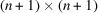International Tables for Crystallography (2006). Vol. A1. ch. 1.2, pp. 6-23   | 1 | 2 | https://doi.org/10.1107/97809553602060000538

## Contents

• 1.2. General introduction to the subgroups of space groups  (pp. 6-23)
• 1.2.1. General remarks  (p. 6) | html | pdf |
• 1.2.2. Mappings and matrices  (pp. 6-9) | html | pdf |
• 1.2.2.1. Crystallographic symmetry operations  (pp. 6-7) | html | pdf |
• 1.2.2.2. Coordinate systems and coordinates  (p. 7) | html | pdf |
• 1.2.2.3. The description of mappings  (pp. 7-8) | html | pdf |
• 1.2.2.4. Matrix–column pairs andmatrices  (p. 8) | html | pdf |
• 1.2.2.5. Isometries  (p. 8) | html | pdf |
• 1.2.2.6. Vectors and vector coefficients  (pp. 8-9) | html | pdf |
• 1.2.2.7. Origin shift and change of the basis  (p. 9) | html | pdf |
• 1.2.3. Groups  (pp. 9-10) | html | pdf |
• 1.2.3.1. Some properties of symmetry groups  (pp. 9-10) | html | pdf |
• 1.2.3.2. Group isomorphism and homomorphism  (p. 10) | html | pdf |
• 1.2.4. Subgroups  (pp. 11-12) | html | pdf |
• 1.2.4.1. Definition  (p. 11) | html | pdf |
• 1.2.4.2. Coset decomposition and normal subgroups  (p. 11) | html | pdf |
• 1.2.4.3. Conjugate elements and conjugate subgroups  (pp. 11-12) | html | pdf |
• 1.2.4.4. Factor groups and homomorphism  (p. 12) | html | pdf |
• 1.2.4.5. Normalizers  (p. 12) | html | pdf |
• 1.2.5. Space groups  (pp. 13-16) | html | pdf |
• 1.2.5.1. Space groups and their description  (p. 13) | html | pdf |
• 1.2.5.2. Classifications of space groups  (p. 14) | html | pdf |
• 1.2.5.3. Space groups and space-group types  (pp. 14-15) | html | pdf |
• 1.2.5.4. Point groups and crystal classes  (pp. 15-16) | html | pdf |
• 1.2.5.5. Crystal systems and crystal families  (p. 16) | html | pdf |
• 1.2.6. Types of subgroups of space groups  (pp. 16-18) | html | pdf |
• 1.2.6.1. Introductory remarks  (p. 16) | html | pdf |
• 1.2.6.2. Definitions and examples  (pp. 16-18) | html | pdf |
• 1.2.6.3. The role of normalizers for group–subgroup pairs of space groups  (p. 18) | html | pdf |
• 1.2.7. Application to domain structures  (pp. 18-22) | html | pdf |
• 1.2.7.1. Introductory remarks  (pp. 18-19) | html | pdf |
• 1.2.7.2. Domains, domain states and symmetry states  (pp. 19-20) | html | pdf |
• 1.2.7.3. Translational domain structures (translation twins)  (pp. 20-21) | html | pdf |
• 1.2.7.4. Domain structures of a general phase transition  (pp. 21-22) | html | pdf |
• 1.2.8. Lemmata on subgroups of space groups  (pp. 22-23) | html | pdf |
• 1.2.8.1. General lemmata  (pp. 22-23) | html | pdf |
• 1.2.8.2. Lemmata on maximal subgroups  (p. 23) | html | pdf |
• References | html | pdf |
• Tables
• Table 1.2.3.1. Multiplication table of a group  (p. 10) | html | pdf |